# Deep Learning with Python¶

In :
%pylab inline
from ipypublish import nb_setup

Populating the interactive namespace from numpy and matplotlib


In this chapter we focus on implementing the same deep learning models in Python. This complements the examples presented in the previous chapter om using R for deep learning. We retain the same two examples. As we will see, the code here provides almost the same syntax but runs in Python. There are very few changes needed, but are the obvious ones for programming differences between the two languages. However, it is useful to note that TensorFlow in Python may be used without extensive knowledge of Python itself. In this sense, packages for implementing neural nets have begun to commoditize deep learning.

Here is the code in Python to fit the model and then test it. Very little programming is needed. First, we import all the required libraries.

import pylab

from keras.models import Sequential
from keras.layers import Dense, Dropout, Activation
from keras import backend
from keras.utils import to_categorical


## Cancer Data¶

Next, we read in the data from the cancer data set we have seen earlier. We also structure the date to prepare it for use with TensorFlow.

#Read in data
import pandas as pd
n = len(tf_train)

X_train = tf_train.iloc[:,1:10].values
X_test = tf_test.iloc[:,1:10].values
y_train = tf_train.iloc[:,10].values
y_test = tf_test.iloc[:,10].values

idx = y_train; y_train = zeros(len(idx));
y_train[idx=='malignant']=1; y_train = y_train.astype(int)

idx = y_test; y_test = zeros(len(idx));
y_test[idx=='malignant']=1; y_test = y_test.astype(int)

Y_train = to_categorical(y_train,2)


The model is then structured. We employ a fully-conected feed-forward network with five hidden layers, each with 512 neurons, Dropout of 25 percent is applied. The node functional form used is Leaky ReLU. The model is compiled as well.

#Set up and compile the model
model = Sequential()
n_units = 512
data_dim = X_train.shape

model.compile(optimizer='rmsprop',
loss='binary_crossentropy',
metrics=['accuracy'])


Finally, the model is fit to the data. We use a batch size of 32, and run the model for 15 epochs.

#Fit the model
bsize = 32
model.fit(X_train, Y_train, batch_size=bsize, epochs=15, validation_split=0.0, verbose=1)


The programming object for the entire model contains all its information, i.e., the specification of the model as well as it's fitted coefficients (weights). In the next section of code, we see how to take the model object and then apply it to the data for testing. We use the scikit-learn package to generate the confusion matrix for the fit. We also calculate the accuracy of the model, i.e., the ratio of the sum of the diagonal elements of the confusion matrix to the total of all its elements.

## OUT-SAMPLE ACCURACY
from sklearn.metrics import confusion_matrix
yhat = model.predict_classes(X_test,batch_size=bsize)
cm = confusion_matrix(y_test,yhat)
print("Confusion matrix = "); print(cm)
acc = sum(diag(cm))/sum(cm)
print("Accuracy = ",acc)


We run the code. Here is a sample of the training run. We only display the first few epochs. See Figure cancertraining.

In :
#cancer_training
nb_setup.images_hconcat(["DL_images/cancer_training.png"], width=600)

Out: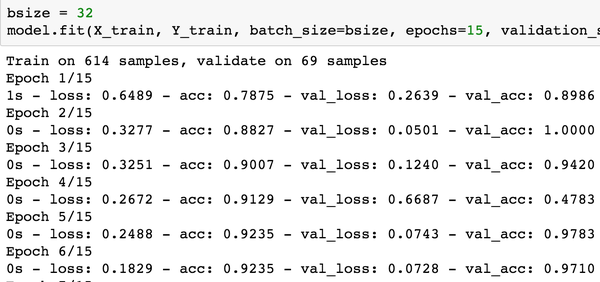Next, is the output from testing the fitted model, and the corresponding confusion matrix. See Figure cancertesting.

In :
#cancer_testing
nb_setup.images_hconcat(["DL_images/cancer_testing.png"], width=600)

Out: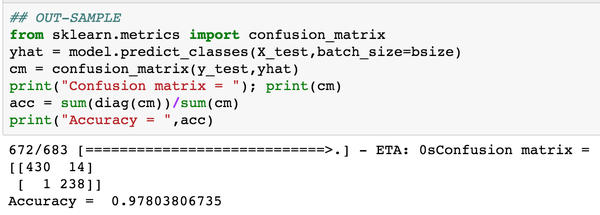## MNIST Data¶

We move on to the second canonical example, the classic MNIST data set. The data set is read in here, and formatted appropriately.

train = pd.read_csv("train.csv")

X_train = train.iloc[:,:-1].values
y_train = train.iloc[:,-1].values
X_test = test.iloc[:,:-1].values
y_test = test.iloc[:,-1].values

Y_train = to_categorical(y_train,10)


Notice that the training dataset is in the form of 3d tensors, of size $60,000 \times 28 \times 28$. (A tensor is just a higher-dimensional matrix, usually applied to mathematica structures that are greater than two dimensions.) This is where the "tensor" moniker comes from, and the "flow" part comes from the internal representation of the calculations on a flow network from input to eventual output.

Each pixel in the data set comprises a number in the range (0,255), depending on how dark the writing in the pixel is. This is normalized to lie in the range (0,1) by dividing all values by 255. This is a minimal amount of feature engineering that makes the model run better.

X_train = X_train/255.0
X_test = X_test/255.0


Define the model in Keras as follows. Note that we have hree hidden layers of 512 nodes each. The input layer has 784 elements.

model = Sequential()
n_units = 512
data_dim = X_train.shape



Then, compile the model.

model.compile(optimizer='rmsprop',
loss='categorical_crossentropy',
metrics=['accuracy'])


Finally, fit the model. We use a batch size of 32, and 5 epochs. We also keep 10 percent of the sample for validation.

bsize = 32
model.fit(X_train, Y_train, batch_size=bsize, epochs=5, validation_split=0.1, verbose=2)


The fitting run is as follows. We see that the training and validation accuracy are similar to each other, signifying that the model is not being overfit. See Figure mnisttraining.

In :
#mnist_training
nb_setup.images_hconcat(["DL_images/mnist_training.png"], width=600)

Out: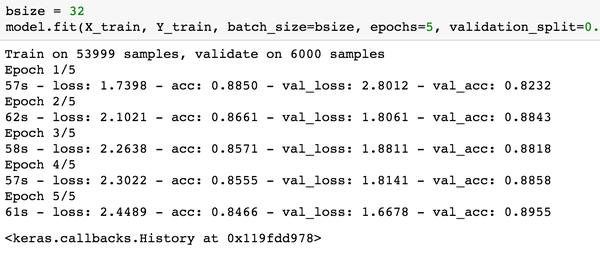Here is the output from testing the fitted model, and the corresponding confusion matrix. See Figure mnisttesting.

In :
#mnist_testing
nb_setup.images_hconcat(["DL_images/mnist_testing.png"], width=600)

Out: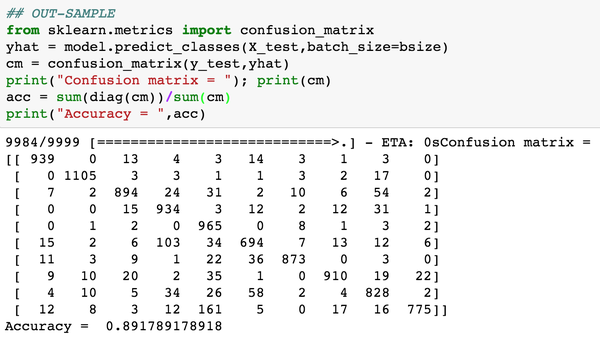The accuracy of the model is approximately 89%. We then experimented with a smaller sized model where the hidden layers only have 100 nodes each (instead of the 512 used earlier). We see a dramatic improvement in the model fit, the out-of-sample fit is shown below. Now, the accuracy is 96%. Therefore, a smaller model does, in fact, do better! Parsimony pays. See Figure mnisttesting2.

In :
#mnist_testing2
nb_setup.images_hconcat(["DL_images/mnist_testing2.png"], width=600)

Out: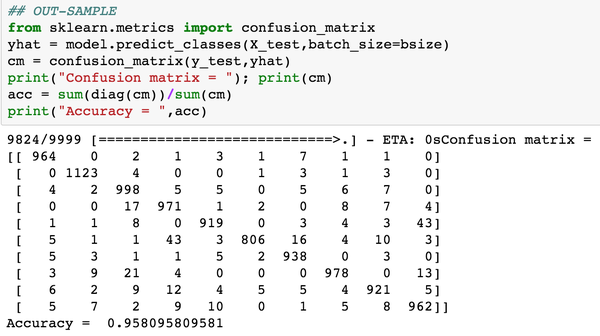## Option Pricing¶

In a recent article, Culkin and Das (2017) showed how to train a deep learning neural network to learn to price options from data on option prices and the inputs used to produce these options prices.

In order to do this, options prices were generated using random inputs and feeding them into the well-known Black and Scholes (1973) model. See also Merton (1973). The formula for call options is as follows.

$$C = S e^{-qT} N(d_1) - K e^{-rT} N(d_2)$$

where

$$d_1 = \frac{\ln(S/K) + (r-q-0.5 \sigma^2)T}{\sigma \sqrt{T}}; \quad d_2 = d_1 - \sigma \sqrt{T}$$

and $S$ is the current stock price, $K$ is the option strike price, $T$ is the option maturity, $q$, $r$ are the annualized dividend and risk-free rates, respectively; and $\sigma$ is the annualized volatility, i.e., the annualized standard deviation of returns on the stock. The authors generated 300,000 call prices from randomly generated inputs (see Table 1 in @CulkinDas), and then used this large number of observations to train and test a deep learning net that was aimed to mimic the Black-Scholes equation. Here is some sample data used for training the model. The first six columns show the inputs and the last column will need to be designed to emit a positive continuous output. See Figure BSNNsampleData.

In :
#BS_NN_sampleData
nb_setup.images_hconcat(["DL_images/BS_NN_sampleData.png"], width=600)

Out: